Find the 10

Find the value of t if 2tx+5y-6=0 and 5x-4y+8=0 are perpendicular, parallel, what angle does each of the lines make with the x-axis, find the angle between the lines?

Result

t1 =  2
A1 =  -38.66 °
A2 =  -128.66 °
t2 =  -3.125
B1 =  51.34 °
B2 =  -128.66 °

Solution:Leave us a comment of example and its solution (i.e. if it is still somewhat unclear...):Be the first to comment!To solve this example are needed these knowledge from mathematics:

For Basic calculations in analytic geometry is helpful line slope calculator. From coordinates of two points in the plane it calculate slope, normal and parametric line equation(s), slope, directional angle, direction vector, the length of segment, intersections the coordinate axes etc. Two vectors given by its magnitudes and by included angle can be added by our vector sum calculator. Most natural application of trigonometry and trigonometric functions is a calculation of the triangles. Common and less common calculations of different types of triangles offers our triangle calculator. Word trigonometry comes from Greek and literally means triangle calculation.

Next similar examples:

1. Reference angleFind the reference angle of each angle:
2. SlopeFind the slope of the line: x=t and y=1+t.
3. LineIt is true that the lines that do not intersect are parallel?
4. Theorem proveWe want to prove the sentence: If the natural number n is divisible by six, then n is divisible by three. From what assumption we started?
5. The angle of lines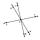Calculate the angle of two lines y=x-21 and y=-2x+14
6. Three workshopsThere are 2743 people working in three workshops. In the second workshop works 140 people more than in the first and in third works 4.2 times more than the second one. How many people work in each workshop?
7. Coordinates of vector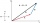Determine the coordinate of a vector u=CD if C(19;-7) and D(-16;-5)
8. LineLine p passing through A[-10, 6] and has direction vector v=(3, 2). Is point B[7, 30] on the line p?
9. TrigonometryIs true equality? ?
10. Vector - basic operationsThere are given points A [-9; -2] B [2; 16] C [16; -2] and D [12; 18] a. Determine the coordinates of the vectors u=AB v=CD s=DB b. Calculate the sum of the vectors u + v c. Calculate difference of vectors u-v d. Determine the coordinates of the vector w.
11. Points collinear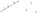Show that the point A(-1,3), B(3,2), C(11,0) are col-linear.
12. VectorDetermine coordinates of the vector u=CD if C[19;-7], D[-16,-5].
13. Vectors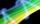For vector w is true: w = 2u-5v. Determine coordinates of vector w if u=(3, -1), v=(12, -10)
14. Scalar product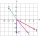Calculate the scalar product of two vectors: (2.5) (-1, -4)
15. Elimination methodSolve system of linear equations by elimination method: 5/2x + 3/5y= 4/15 1/2x + 2/5y= 2/15
16. Sequence 2Write the first 5 members of an arithmetic sequence a11=-14, d=-1
17. BlocksThere are 9 interactive basic building blocks of an organization. How many two-blocks combinations are there?